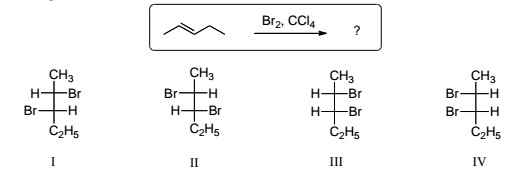# Problem: Which of the statements is most correct regarding the products expected from the halogenation reaction shown below? a) Equal amounts of I and IV are produced. b) Equal amounts of I and III are produced. c) Equal amounts of III and IV are produced. d) Equal amounts of I and II are produced. e) Equal amounts of II and III are produced.

###### FREE Expert Solution
92% (253 ratings)
###### Problem Details

Which of the statements is most correct regarding the products expected from the halogenation reaction shown below?

a) Equal amounts of I and IV are produced.

b) Equal amounts of I and III are produced.

c) Equal amounts of III and IV are produced.

d) Equal amounts of I and II are produced.

e) Equal amounts of II and III are produced.What scientific concept do you need to know in order to solve this problem?

Our tutors have indicated that to solve this problem you will need to apply the Halogenation concept. You can view video lessons to learn Halogenation. Or if you need more Halogenation practice, you can also practice Halogenation practice problems.

What is the difficulty of this problem?

Our tutors rated the difficulty ofWhich of the statements is most correct regarding the produc...as high difficulty.

How long does this problem take to solve?

Our expert Organic tutor, Chris took 2 minutes and 15 seconds to solve this problem. You can follow their steps in the video explanation above.

What professor is this problem relevant for?

Based on our data, we think this problem is relevant for Professor Rodriguez's class at SPELMAN.